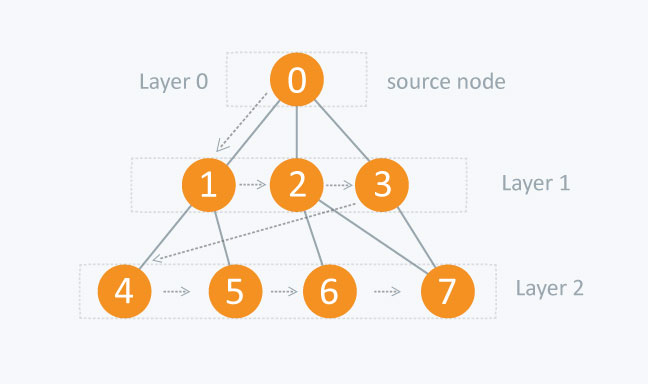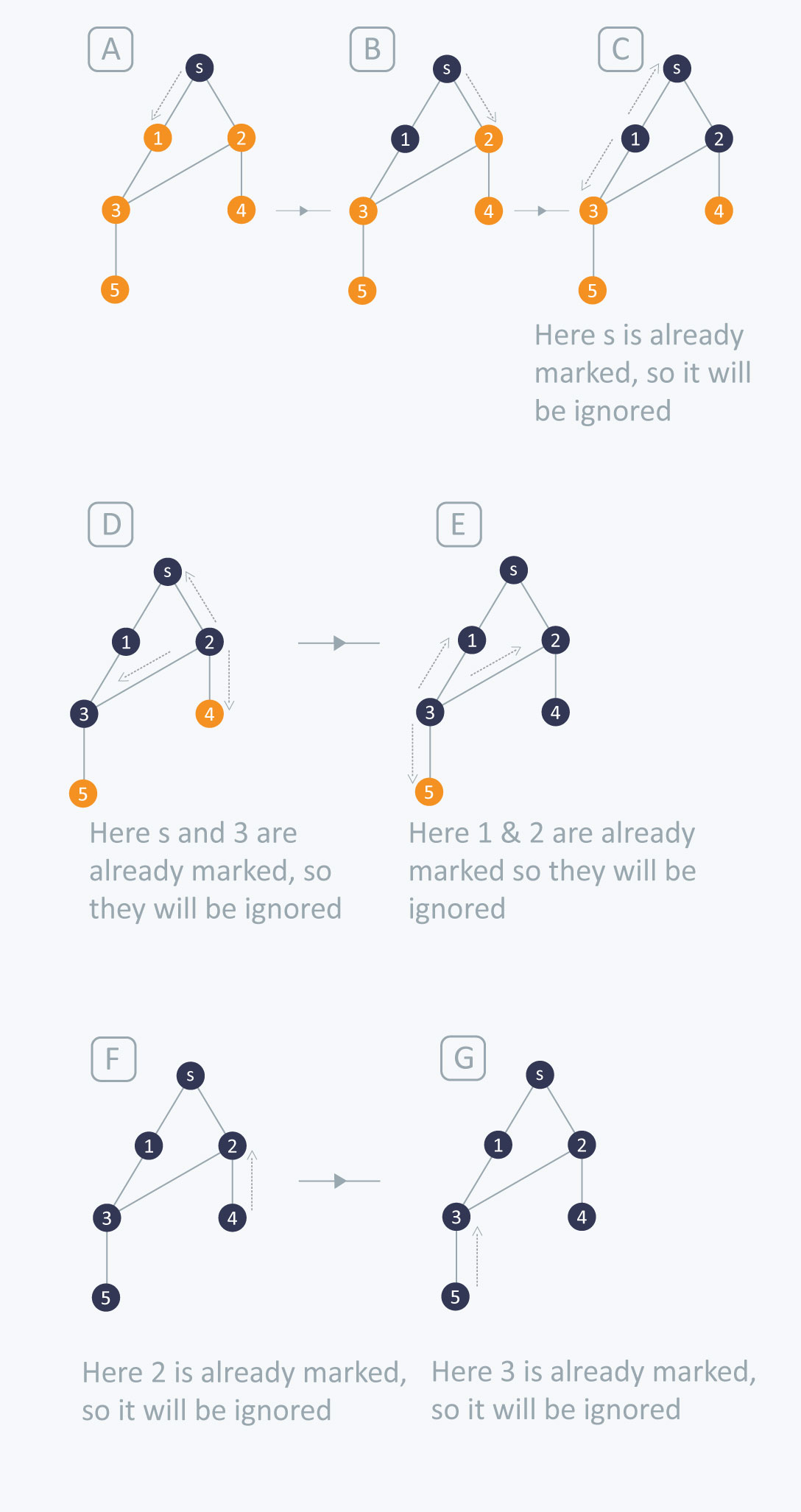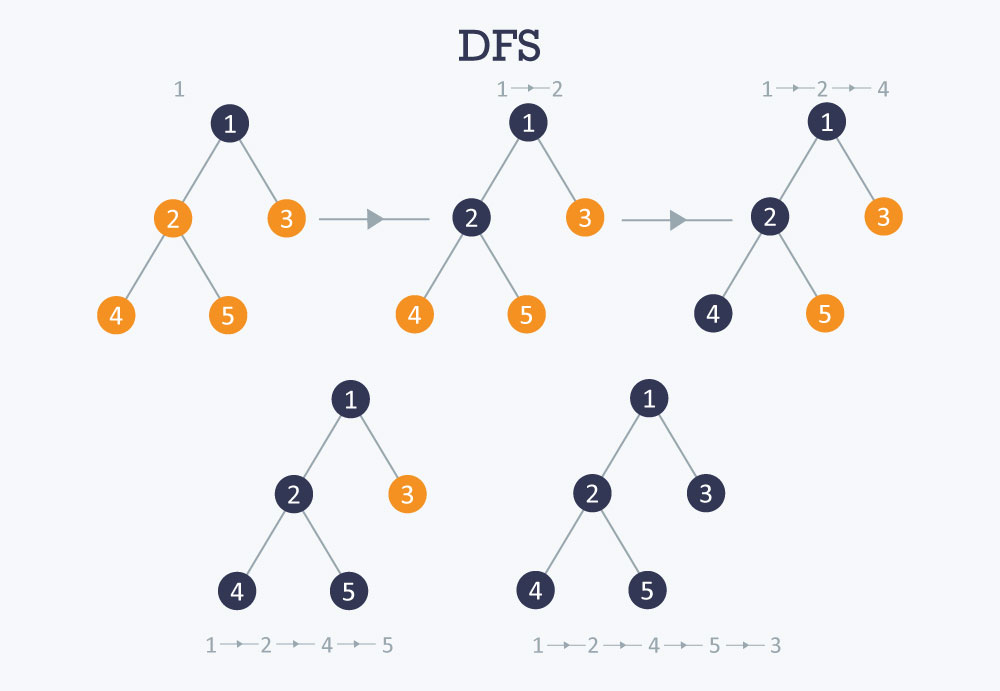# ALGORITHM

## 1.Solved algorithm for BFS Algorithm design analysis

 Best sorting and searching method in daaAlgorithm design analysisAlgorithm procedue

The traversing will start from the source node and push s in queue. s will be marked as ‘visited’.

## First iteration

• s will be popped from the queue
• Neighbors of s i.e. 1 and 2 will be traversed
• 1 and 2, which have not been traversed earlier, are traversed. They will be:
• Pushed in the queue
• 1 and 2 will be marked as visited

## Second iteration

• 1 is popped from the queue
• Neighbors of 1 i.e. s and 3 are traversed
• s is ignored because it is marked as ‘visited’
• 3, which has not been traversed earlier, is traversed. It is:
• Pushed in the queue
• Marked as visited

## Third iteration

• 2 is popped from the queue
• Neighbors of 2 i.e. s, 3, and 4 are traversed
• 3 and s are ignored because they are marked as ‘visited’
• 4, which has not been traversed earlier, is traversed. It is:
• Pushed in the queue
• Marked as visited

## Fourth iteration

• 3 is popped from the queue
• Neighbors of 3 i.e. 1, 2, and 5 are traversed
• 1 and 2 are ignored because they are marked as ‘visited’
• 5, which has not been traversed earlier, is traversed. It is:
• Pushed in the queue
• Marked as visited

## Fifth iteration

• 4 will be popped from the queue
• Neighbors of 4 i.e. 2 is traversed
• 2 is ignored because it is already marked as ‘visited’

## Sixth iteration

• 5 is popped from the queue
• Neighbors of 5 i.e. 3 is traversed
• 3 is ignored because it is already marked as ‘visited’

The queue is empty and it comes out of the loop. All the nodes have been traversed by using BFS.

If all the edges in a graph are of the same weight, then BFS can also be used to find the minimum distance between the nodes in a graph.

## 2. Algorithm for DFSAlgorithm procedure

Algorithm procedure formula solving problem

Time complexity O(V+E), when implemented using an adjacency list.

## How to find connected components using DFS?

Algorithm procedure

A graph is said to be disconnected if it is not connected, i.e. if two nodes exist in the graph such that there is no edge in between those nodes. In an undirected graph, a connected component is a set of vertices in a graph that are linked to each other by paths.

Consider the example given in the diagram. Graph G is a disconnected graph and has the following 3 connected components.

• First connected component is 1 -> 2 -> 3 as they are linked to each other
• Second connected component 4 -> 5
• Third connected component is vertex 6

In DFS, if we start from a start node it will mark all the nodes connected to the start node as visited. Therefore, if we choose any node in a connected component and run DFS on that node it will mark the whole connected component as visited.

Input File
6
4
1 2
2 3
1 3
4 5

## 3.Dijkstra’s algorithm:

Dijkastra algorithm is step by step process to find out shortest path between two vertices in a weighted graph.

## Shortest Path Problem

Have you ever used Google Maps to find the shortest route or minimum cost of gas to get from one point to another? If so, then you’ve encountered an example of the shortest path problem. In math terms, this is a way to find the shortest possible distance between two vertices on a graph.

Suppose we’re trying to find the shortest path from your house to Divya’s house. We know the distances between various locations throughout town. If we let various locations be vertices and the routes between them be edges, we can create a weighted graph representing the situation. Quick definition: a weighted graph is a collection of vertices and edges with edges having a numerical value (or weight) associated with them.

The graph is good example for weighted graph,there are different paths but we select that path which are shortest path.

## Dijkastra’s Algorithm:

Dijkstra’s algorithm is an algorithm we can use to find shortest distances or minimum costs depending on what is represented in a graph. You’re basically working backwards from the end to the beginning, finding the shortest leg each time. The steps to this algorithm are as follows:

Step 1: Start at the ending vertex by marking it with a distance of 0, because it’s 0 units from the end. Call this vertex your current vertex, and put a circle around it indicating as such.

Step 2: #Identify all of the vertices that are connected to the current vertex with an edge. Calculate their distance to the end by adding the weight of the edge to the mark on the current vertex. Mark each of the vertices with their corresponding distance, but only change a vertex’s mark if it’s less than a previous mark. Each time you mark the starting vertex with a mark, keep track of the path that resulted in that mark.

Step 3: Label the current vertex as visited by putting an X over it. Once a vertex is visited, we won’t look at it again.

Step 4: Of the vertices you just marked, find the one with the smallest mark, and make it your current vertex. Now, you can start again from step 2.

Step 5: Once you’ve labeled the beginning vertex as visited – stop. The distance of the shortest path is the mark of the starting vertex, and the shortest path is the path that resulted in that mark.

Let’s now consider finding the shortest path from your house to Divya’s house to illustrate this algorithm.

## Application

First, we start at the ending vertex (Divya’s house). Mark it with a zero and call this vertex the current vertex, putting a circle around it, as you can see on the graph:

The next step is to mark any vertices that are connected to Divya’s house by an edge with the distance from the end. Let’s quickly calculate these distances while we look at a visual representation of what’s going on using our previous graph.

• Movie theater = 0 + 4 = 4
• Grocery store = 0 + 5 = 5
• Gas station = 0 + 6 = 6

We’re now done with Divya’s house, so we label it as visited with an X. We see that the smallest marked vertex is the movie theater with a mark of 4. This our new current vertex, and we start again at step 2.

## 1.Divide-and-conquer

The 2 sorting algorithm, selection sort and insertion sort, have worst-case running times of Θ (n2).when size of problem is large and take large time to solve. we having two sorting technique, merge sort and quicksort, whose running times are better. In particular, merge sort runs in Θ(nlgn) time in all cases, and quicksort runs in Θ (n \lg n) Θ(nlgn) time in the best case and on average, though its worst-case running time is Θ(n^2) Θ(n2 ). There are 4 sorting algorithm with runing time:

AlgorithmWorst-case
time
Best-case
time
Average-case
time
Selection sort
Θ (n^2)Θ(n2)

Θ (n^2)Θ(n2)

Θ (n^2)Θ(n2)
Insertion sort
Θ (n^2)Θ(n2)

Θ (n)Θ(n)

Θ (n^2)Θ(n2)
Merge
sort

Θ (n \lg n)Θ(nlgn)

Θ (n \lg n)Θ(nlgn)

Θ (n \lg n)Θ(nlgn)
Quick
sort
Θ (n^2)Θ(n2)Θ (n \lg n)Θ(nlgn) Θ (n \lg n)Θ(nlgn)

If we expand out 2 recursive steps, so it look like this:

Divide-and-conquer
Merge sort and Quick sortemploy a common algorithmic paradigm based on recursion. Now there use technique divide-and-conquer, breaks a problem into subproblems respectivly and solve all ther subproblem one by one ,whenever you solve all sub problems you should conquer all the problem and you will find answer of that problem.
Divide and Conqur are 3 points:
Divide the problem into subproblems.
Conquer the subproblems by solving them recursively. If they are small enough, solve the subproblems as base cases.
Combine the solutions to the subproblems and obtained real result.
you can easly remember the step of this sorting tecnique

## 2. Quick Sort Algorithm

QuickSort is one of the most efficient sorting algorithms and is based on the splitting of an array into smaller ones. The name comes from the fact that, quick sort is capable of sorting a list of data elements significantly faster than any of the common sorting algorithms. And like Merge sort, Quick sort also falls into the category of divide and conquer approach of problem-solving methodology.

Quick sort works in the following manner:

Taking the algorithm view :
Select a splitting value, say L. The splitting value is also known as Pivot.
Divide the stack of papers into two. A-L and M-Z. It is not necessary that the piles should be equal.
Repeat the above two steps with the A-L pile, splitting it into its significant two halves. And M-Z pile, split into its halves. The process is repeated until the piles are small enough to be sorted easily.
Ultimately, the smaller piles can be placed one on top of the other to produce a fully sorted and ordered set of papers.
The approach used here is recursion
At every split, the pile was divided and then the same approach was used for the smaller piles.
Due to these features, quick sort is also called as exchange sort.
example:
The following array: {9, 7, 5, 11, 12, 2, 14, 3, 10, 6}

## 3.Marge Sort

Marge sort is top-down approches
it will follow divide and conqure technique to solve problem.
Example: Let us consider an example for better understanding.

## 4. Bubble Sort

Bubble Sort is simple algorithm we have “n” numbers pf elements. bubble sort compare elements one by one.

if given arry has to be sort in ascending order , then this algorithm will start comparing from first element of the array with the second element if first element is greater then secound element so it will swap both elements, and then move on to compare the second and the third element, and so on.

Implementing Bubble Sort Algorithm

Following are the steps involved in bubble sort

1. Starting with the first element(index = 0), compare the current element with the next element of the array.
2. If the current element is greater than the next element of the array, swap them.
3. If the current element is less than the next element, move to the next element. Repeat Step 1.

Let’s consider an array with values `{5, 1, 6, 2, 4, 3}`

Below, we have a pictorial representation of how bubble sort will sort the given array.

So as we can see in the representation above, after the first iteration, `6` is placed at the last index, which is the correct position for it.